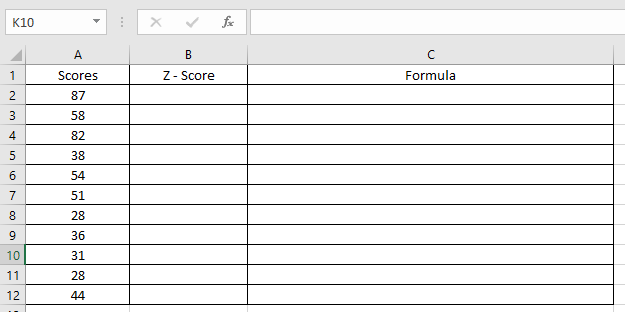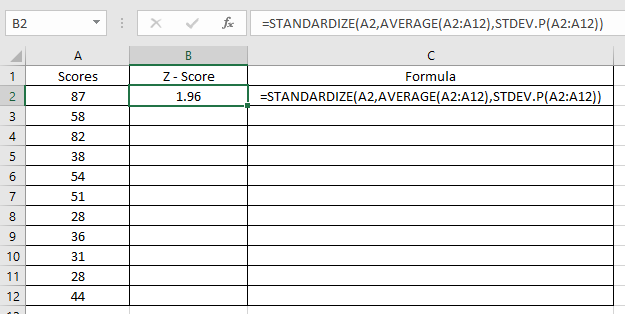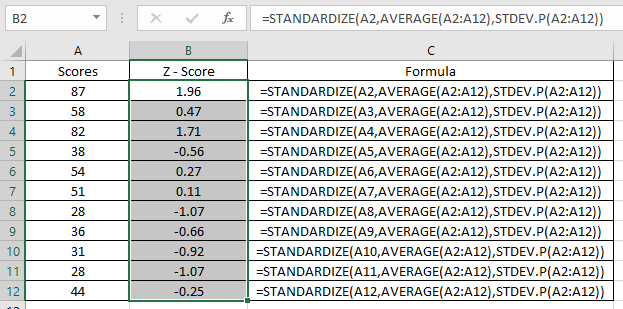# How to use the Excel STANDARDIZE Function

To get the normalised score (Z-score) of the value in the data set having mean (Average) and standard daviation of the data, we use the below formula in mathematics.

Z-score = (Value - Average) / (Standard deviation)

The STANDARDIZE function is a statistical function function. It takes returns a normalized value (z-score) for the values of the data
Syntax:

=STANDARDIZE (value, mean, standard_deviation)

Value : value to be normalised
Mean : Average of values
Standard_deviation : Standard deviation of the data
Let’s understand this function using it in an example.Here we have scores of the students and we need to find the Z score for the data.

For mean, we will use the AVERAGE function and For Standard deviation, STDEV.P function.

Use the formula:

=STANDARDIZE(A2, AVERAGE(A2:A12), STDEV.P(A2:A12))

A2 : value to be normalised
AVERAGE(A2:A12) : mean of the data
STDEV.P(A2:A12) : Standard deviation of the dataAs you can see the Z-score and its formula in adjacent cell from the above snapshot.

Copy the formula in other cells using shortcut key Ctrl + DAs you can see the normalised value (Z-score) for the data.

Hope you understood how to use STANDARDIZE function in Excel. Explore more articles on Excel function here. Please feel free to state your query or feedback for the above article.

Related Articles:

How to Calculate Mean in Excel

How to Use STDEV Function in Excel

How to Create Standard Deviation Graph in Excel

Popular Articles:

How to use the VLOOKUP Function in Excel

How to use the COUNTIF function in Excel 2016

How to Use SUMIF Function in Excel

Terms and Conditions of use

The applications/code on this site are distributed as is and without warranties or liability. In no event shall the owner of the copyrights, or the authors of the applications/code be liable for any loss of profit, any problems or any damage resulting from the use or evaluation of the applications/code.